# The Magic of Algebra

I must apologize. Science4All’s summer was unusually calm, with no new entry in 3 months. But I have a good excuse: I spent my summer actively working on two 1-hour video documentaries of undergrad mathematics entitled: “La magie des maths de prépa”, which roughly translates into “The magic of undergrad maths” (more about “prépas” here). Unfortunately for my non-French readers, the documentary is in French, although there are English subtitles. Here’s its trailer.

In this article, I’ll retell the first episode on algebra, although I will remove/add stuffs to the story-telling to consolidate the Historical account.

## Al-jebr

Students often complain about having to learn algebra. Why should we use symbolic letters to represent numbers when, eventually, we’ll replace these letters by numbers anyway? They’re not the only one to think so. For thousands of years, the greatest minds didn’t waste time representing abstractly the numbers they were thinking about. Yet, as counter-intuitive as it may sound, this is precisely the reason why they never got far in their understanding of numbers. In particular, every new problem involving new numbers was precisely that: A new problem. But then came Al-Khwarizmi.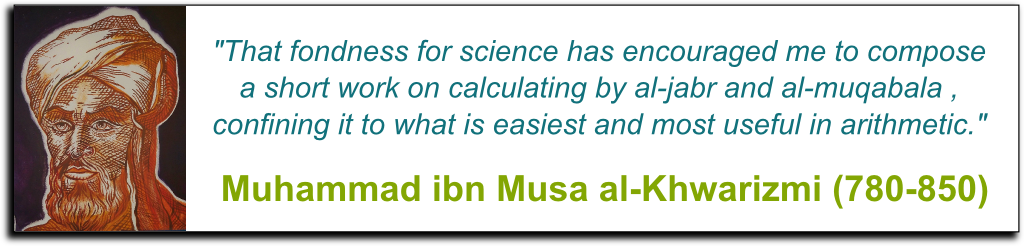Who’s Al-Khwarzimi?

Al-Khwarizmi was a member of Baghdad’s House of Wisdom. In the heart of the young Islamic civilization, Baghdad was a center of business. Travellers were coming from East and West with new goods to trade. Among these goods were the gifts of science and mathematics. And Al-Khwarzimi was one of the Islamic scholars that took advantage of this privileged situation to advance the frontier of knowledge. But Al-Khwarizmi was not just any scholar. He was a genius. And he brilliantly unified all knowledge of numbers into a new formalism that we still use today: Al-jebr.

Al-jebr? Don’t you mean algebra?

Al-jebr literally translates into “the reunion of broken parts”. This is a nice way of describing mathematics: We often aim at connecting apparently disconnected pieces. Yet, beyond this epistemological aspect, Al-Khwarizmi’s breakthrough was to introduce a revolutionary method to address problems.

Which is…

In essence, the key aspect of Al-Khwarizmi’s al-jebr is its systematic use of abstraction to generalize patterns. It might sound surprising at first to think that abstraction is the unavoidable path to generalization. After all, “to abstract” basically means “to forget”. But what does forgetting have to do with generalization?

Hummm… That’s puzzling…

Well, here’s the example I used in the documentary to illustrate that, where, in essence, I explain that weighing scale problems with apples are absolutely not problems of apples:

In some sense, in this example, it’s helpful to forget that the thing we’re trying to weigh is an apple.

That’s pretty obvious, isn’t it?

Then let’s take a more sophisticated example. Let’s consider the equation $?-1=1$. It is easy to see that $2$ is the solution of this equation. Al-Khwarizmi’s key insight is to generalize any such equation by forgetting, for instance, that this equation involves the numbers $1$.

What does that mean?

Well, roughly, forgetting that $1$s appear in this equation may correspond to rewrite the equation $?-*=*$. Now, I don’t know about you, but I think that there starts to be too many confusing symbols in my equation. This is because the language I’m using is inconvenient. The genius of Al-Khwarizmi was not only to realize that there is a huge potential in forgetting all sorts of stuffs, but also to devise a language to do so. This language is al-jebr, which in modern English is written algebra.

How does this work?

To forget that $1$ is $1$, it suffices to replace it by an abstract symbol like a symbolic letter, say $a$. Replacing $1$ by $a$ leads to equation $?-a=a$. Interestingly, the language of algebra also enables us to notice that the two $1s$ were not necessarily the same $1s$, and we might want to distinguish them. Thus, we may use another symbolic letter, say $b$, for the latter $1$. This yields the equation $?-a=b$. At last, the power of abstraction and the language of algebra have enabled us to generalize our problem!

Oh yeah, by the way, we don’t use the symbol $?$ to represent unknowns anymore. We use the symbol $x$. This is because Al-Khwarizmi used the word shin, which means something. This word has then been loosely translated to eventually become the letter $x$. Here’s one great TedTalk about that by Terry Moore:

In particular, Al-Khwarizmi’s willingness to forget and his language of algebra enabled him to state and solve first degree equations $ax+b=c$ and second degree equations $ax^2+bx+c=0$. I’m sure you’ve already seen these equations, and they’ve probably even repelled you away from mathematics. Fortunately, mathematics in general, and algebra in particular, have evolved since to address many questions that are much more interesting!

But at the core of any modern mathematics, there are still Al-Khwarzimi’s stubborn willingness to forget and his powerful language of algebra.

## Cubic and Quartic Equations

Unfortunately, Al-Khwarizmi’s breakthrough might have been too advanced for his contemporary scholars to build upon. Even worse, the House of Wisdom was destroyed after Baghdad’s fall in 1258 against the huge Mongolian empire. His marvellous ideas nearly died. But, fortunately, European scholars picked them up. Although, it did take them a while. But in the 16th century, Tartaglia, Cardano and Ferrari made a breakthrough that really naturally extended Al-Khwarizmi’s ideas.

What did they do?

The story of these geniuses is a marvellous story of rivalries and deceptions – the kind of stories that’d deserve a Hollywood movie! In those days, mathematicians often had public mathematical duals.

What?

Well, you know. Two mathematicians would stand in front of each other. A third person would challenge them with a mathematical question. And, well, the first to shoot rightly would win the dual.

That sounds…

I know! It sounds so freaking awesome!

I was going to say geek…

Hummm… I see what you mean… Anyways, some of the usual challenges were to solve cubic equations, like $x^3-2x^2+5x=24$. And at some point, Tartaglia started to become undefeated. Worse, he appeared unbeatable. Why? Weirdly enough, that wasn’t because he was particularly smart. Rather, he became unbeatable, because he had hacked all dual challenges at once!

What do you mean?

Following Al-Khwarizmi’s insight, Tartaglia forgot about the particular digits of the equation. He faced, and solved, the generalized cubic equation $ax^3+bx^2+cx=d$. But he kept it secret, maybe because he wanted to carry on his winning strike. And this worked out brilliantly! However, at some point, Cardano learned about Tartaglia’s clever generalized solution. Tartaglia swore him to secrecy. Cardano accepted. At first.

Did Cardano betray him?

The thing is that, by building upon Tartaglia’s ideas, Cardano’s student Ferrari also cracked quartic equations of the form $ax^4+bx^3+cx^2+dx=e$. Cardano probably felt uncomfortable barring Ferrari’s breakthrough for a secrecy he had been sworn about a simpler problem than Ferrari’s. Maybe he also realized the importance of such breakthroughs in the History of mathematics and science. Or perhaps he was threatened by Scipione del Ferro’s unpublished independent book. In any case, Cardano ended up breaking the promise he made to Tartaglia, as he published, for the first time in History, the solution to cubic and quartic equations.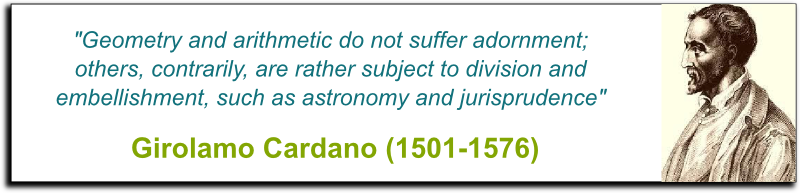Did he at least give credit to Tartaglia?

He did. And to Ferrari as well. But, importantly, Tartaglia was no longer the king of duals. Ferrari had become better. For sure, solving quartic equations gave him additional insights to solve cubic equations even faster. When challenged by Ferrari, Tartaglia did not show up. Tartaglia became discredited. He died poor, sad and forgotten. Today, the solution of cubic equation is known as Cardano’s. Not Tartaglia’s.

## Geometry

As often in mathematics, the first extensions of Al-Khwarizmi’s ideas were easy extensions. Thanks to Tartaglia, Cardano and Ferrari, all equations of degree at most 4 could now be solved (although it requires an uncomfortable trick which we’ll get back to later). But, as often in mathematics, in the longer run, great ideas like Al-Khwarizmi’s lead to unexpected extensions.

What are you hinting at?

I’m hinting at one of the most important unification in the History of mathematics, due to Frenchman mathematician René Descartes.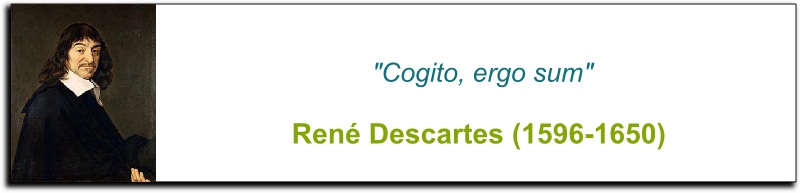What’s this unification?

Basically, at Descartes’ time, there were two kinds of mathematics. There was Euclid’s geometry and Al-Khwarizmi’s algebra. The mind-blowing realization of Descartes was that these were merely two sides of a same coin. More precisely, by introducing a system of coordinates in a plane, points of the plane could now be located by two numbers, often called $x$ and $y$. Moreover, Descartes showed that there was a natural dictionary between geometrical shapes and algebraic equations.

For instance, the ellipses, parabolas and hyperbolas discovered in Antiquity by Archimedes now correspond to second degree equations with two variables of the form $ax^2+bxy+cy^2+dx+ey+f=0$. A posteriori, it’s quite amazing to think that Archimedes’ geometrical shapes correspond exactly to the simplest kinds of nonlinear equations of 2 variables. Well, at least, that’s what I think.

It kind of is!

I know! Thank you! More generally, the union between geometry and algebra is called… well we’re not that imaginative, so we called it algebraic geometry! Amazingly, algebraic geometry asserts that algebraic equations can be solved by focusing on geometrical questions, and vice-versa. And this often helps! For instance, you can check my proof that the primitive of the 1/x is the logarithm, by studying properties of hyperbolas.

But is algebraic geometry still useful to mathematicians nowadays?

Actually, it’s more useful than ever. Since second degree equations are now well understood, mathematicians are currently studying cubic equations, like $y^2=x^3+ax+b$. These are called elliptic curves (although they shouldn’t be, as they have little to do with ellipses!).

Hummm… I’m a bit disappointed, all of sudden…

The thing is that quartic equations are just too hard to analyze. At least so far. Still, as will discuss in a bit, cubic equations already yield wonderful properties that are still not well understood, and that have deep and surprising consequences! But to fully understand them, as weird as it sounds, we must also solve them for numbers other than real numbers. For a start, their solutions in the complex plane are much easier to describe. But also, their solutions for rational numbers, extensions of rational numbers, finite fields or p-adic fields are even more interesting and insightful to understand the properties of all numbers (and this leads some geometers to claim that this no longer has much to do with geometry…). I know I sound unintelligible. But bear with me, I’ll get back to that in a bit!

## Numbers

Back then, René Descartes had a great rival. He was in contention with another genius for the honorific title of France’s greatest genius.

Who’s this other genius?

This other genius is Pierre de Fermat. While Descartes was planting the seeds of algebraic geometry, Fermat was planting those of algebraic number theory, to prove the beautiful hidden patterns of numbers.

What kind of pattern?

Here’s one example: Any prime is the sum of two squares if and only if the remainder of its division by 4 is 1. This is a splendid result because it is totally unexpected. Why on earth would the property of being a sum of two squares be related to divisions by 4? Yet, if you look at this property for small primes, you’ll see that it holds. For instance, $5 = 1^2+2^2$=$4+1$. Using Al-Khwarizmi’s language and willingness to forget about, among other things, the specific values of the primes, Fermat managed to prove that the property also holds for the infinite set of all primes!

Hummm… But of what’s the point in proving that?

Number theorists usually don’t care about the applications of the patterns in numbers they find. Noticing patterns suffices to satisfy their intellect. After all, there is beauty in unveiling true patterns in the everlasting collection of numbers. Nevertheless, applications of the patterns they find sometimes come up unexpectedly.

Really?

Yes! For instance, Fermat also noticed that if you take any prime $p$ and any number $a$, then the remainder of the division of $a^p$ by $p$ is always $a$. For centuries, numbers theorists have been pleased by the mere truth of this fact, now known as Fermat’s little theorem. Needless to say that it seemed far removed from any kind of application…

But?

But in the 1970s, Ron Rivest, Adi Shamir and Leonard Adleman found a way to use these hidden patterns in numbers to design cryptography system, now known as RSA. Today, the RSA system is widely used by banks and the Web, to make sure that private information remains private, and that monetary transfers do not get intercepted.

But Fermat’s most puzzling theorem is yet another one. At some point, he claimed that the equation $x^n+y^n=z^n$ had no integer solutions for $n \geq 3$. Today, this claim is known as Fermat’s last theorem (or maybe, rather, as the Fermat-Wiles theorem). This problem is particularly special in the History of mathematics, because of the wonderful story behind it (enough to make another Hollywood movie, in addition to the already existing BBC documentary?).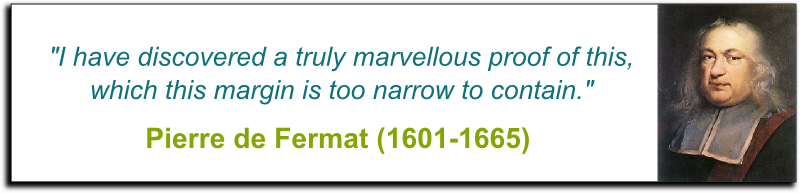As the story goes, Fermat claimed he had a proof, but did not make the effort to write it down. For 350 years, the greatest mathematicians, including Euler, Gauss, Poincaré and others, have tried to follow Fermat’s success. They failed. They all failed. It was only recently, in 1994, totally out of the blue, that Andrew Wiles, after seven years of lonely and secret work and a flawed attempt, finally met Fermat’s challenge and gave a complete proof of his last theorem.

Did Fermat really have a proof?

The poetical answer is that we do not know and shall never know. More pragmatically, most mathematicians now think that he had a wrong proof. After all, the only known proof today builds upon centuries of mathematical developments and was definitely not within Fermat’s reach. In particular, Wiles’ proof makes great use of recent advancements in our understanding of elliptic curves. But Descartes and Fermat’s algebras were still nowhere near compared to the Revolution that was to come.

## Fundamental Theorem of Algebra

Before getting to the Revolution I’m hinting at, I need to get back to Tartaglia, Cardano and Ferrari’s successful resolutions of cubic and quartic equations. Amazingly, they found out that the simplest way to solve these equations was to introduce an illegal trick.

What do you mean by illegal?

I mean that at some point of their computations, they involved a so-called imaginary number. This imaginary number did not appear to exist. But, somehow, it did a wonderful job in simplifying computations, and would disappear eventually anyways!

Why would this number be imaginary?

Well, it’s $\sqrt{-1}$, the number whose square is -1. Now, that’s weird, because squares are usually nonnegative. For instance, $(-1)^2=1>0$. In fact, any of our usual so-called real numbers cannot be that square root of -1. So, whatever $\sqrt{-1}$ is, it is definitely not a real number.

Naturally, I’m using the words “imaginary” and “real” as they would be used back then. But the reason why what I’m saying seems to make sense is mainly because of my use of these words. But, as often in mathematics, it’s important not to be misled by poor terminology! In fact, as ViHart explains it wonderfully, real numbers aren’t that “real”…
So how should I think about imaginary numbers?

For me, the key insight to grasp imaginary numbers is to forget that real and imaginary numbers are “numbers”. The best way to forget this is to involve the concept of fields, which we’ll get to in a bit. But for now, let’s say it like this: Numbers are solutions to equations. Keep this in mind, and forget anything else you’ve ever learned about numbers! Forget that they count, and forget that they are ordered. Amazingly, when you only think of numbers as solutions of equations, the combinations of real and imaginary numbers, called complex numbers, are now obviously the best kinds of numbers.

Why?

The reason for that boils down to what might be the most beautiful fact of algebra. It was proved by Carl Friedrich Gauss, also known as the Prince of mathematics, and who’s often regarded as the greatest mathematician of all times.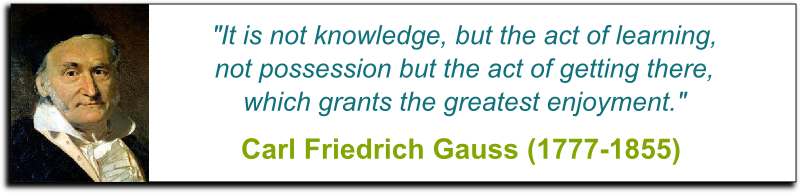So what did Gauss prove?

What Gauss proved was the fundamental theorem of algebra. This theorem asserts that, while some equations like $x^2=-1$ have no real number solution, all of them have solutions complex number solutions (with the exception of stupid equations like $0x+1=0$). In modern mathematical terminology, we say that the set of complex numbers is algebraically closed. Importantly, this means that complex numbers are unbelievably simpler than real numbers when it comes to solving equations. (And in fact, they turn out to be unbelievably simpler when it comes to calculus as well…)

Gauss even gave 3 different proofs of this fact. However, his first proof turned out to be mistaken (and only completed in 1920 by by Alexander Ostrowski), and his second proof was preceded by Jean-Robert Argand’s proof. So Argand was actually the first to give a complete and correct proof of the fundamental theorem of algebra. But, probably because he was merely an amateur while Gauss is the big gun of mathematics, Argand’s success is often eclipsed by Gauss’ grandeur… Even in my articles!

## Modern Algebra

The great French revolution was in 1789, but monarchy came back after Napoleon’s fall in 1815. Young Frenchman Évariste Galois didn’t like that. In 1830, he got jailed for inciting a new Revolution. Next, for obscure reasons, he got challenged to a duel in 1832, which he lost. He died at the age of 20 years old.

If there’s one Hollywood-movie-worth story in the History of mathematics, it’s definitely Galois’. Politics, betrayal, duals, imprisonment, rejection, anger, gun fires, genius and death. All were part of Galois’ short life…

What does that have to do with mathematics?

Evidently, politics was not the only established order Galois wanted to fight. Galois upset our whole understanding of mathematics. For the first time in History, Galois didn’t envision mathematics as the study of numbers or curves. He saw it as the study of structures. This amazing insight has revolutionized mathematics since, not only by blowing up the range of applicability of mathematics, but also by deepening our understanding of numbers and curves as well.

Can you give an example?

For instance, one triumph of Galois theory was to prove the end of Al-Khwarizmi, Tartaglia, Cardano and Ferrari’s run at solving more and more complicated equations: Galois proved that quintic equations could not be solved, at least not by using the usual algebraic operations and radicals. And Galois’ exquisite proof lies in the systematic study of structures, rather than numbers.

What do you mean by structures?

Once again, the key to understand Galois’ insight is abstraction. Following Galois’ ideas, we should forget about the classical meaning we give to numbers. Forget that they count. Forget that some numbers can approximate others. Forget that they are ordered. Instead, focus merely on how numbers interact instead. Considering solely how they add, subtract, multiply, divide and equal leads us to regard the set of numbers as a so-called field. So for instance, rational numbers form a field, because they can be added, subtracted, multiplied and divided, while still producing rational numbers. Similarly, real numbers form a field. And complex numbers too. But there are many other fields!

Like what?

For instance, the set of numbers modulo $p$, when $p$ is prime, is also a field with a finite number of elements! Galois even went on constructing other fields, including finite field extensions of rational numbers like $\mathbb Q[\sqrt{2}]$ and finite field extensions of the set of numbers modulo a prime $p$, whose cardinals are $p^n$. Later, in the late 19th century, it was discovered that these finite fields have a sort of limit when $n$ goes to infinity, which is a field called the field of $p$-adic numbers. All these numbers solved equations. But more importantly, Galois’ abstraction yields a natural definition for numbers: They are elements of a field.

This is not actually right. Indeed, I doubt that anyone sees the field of fractions of polynomials as a set of numbers. I guess that a slightly better definition of numbers is to see them as elements of fields that are not functions. But then, I’m also not considering ordinal and cardinal numbers that are called so mainly because they seem to be “counting”, although what they count are infinite sets… Overall, numbers are called such mainly because of Historical reasons, and no rigorous definition seems necessary.

But Galois went even further in his abstraction. He went on considering structures with no multiplication nor division, but only addition and subtraction. Even better, he forgot about some properties commonly satisfied by addition. At last, this led him to treat complicated objects in the same way that he’d treat to numbers.

What complicated objects do you have in mind?

Symmetries! Just like numbers, Galois noticed that symmetries can be combined. For instance, we may combine a rotation followed by an axial symmetry. Galois wrote this so-called composition of symmetries in almost the same way as we would write an addition of numbers.

Wait… You can’t really compose symmetries exactly as you add numbers, can you?

We almost can. There’s one tricky detail though, called the non-commutativity of symmetries. This is explained below by Marcus du Sautoy, in a Ted Talk:

Compare the composition of rotation then axial symmetry to that of axial symmetry then rotation. You’ll see that these usually don’t match. In other words, the order of composition makes a difference. We say that symmetries form a non-commutative group. Yet, because symmetries are so ubiquitous in Nature and societies, the study of non-commutative groups turned out to be just as fruitful as that of commutative groups like numbers. In particular, the comparison of groups of symmetries led Galois to even more important concepts of mathematics.

Like what?

Galois noticed that some groups defined in different manners were essentially the same. For instance, the symmetries of an equilateral triangle are somehow the same group as the group of permutations of three elements. Nowadays, we’d say that these groups of isomorphic, which is probably today’s most important concept of mathematics. Take any group. Forget about how it is defined. Forget about it is interpreted. Forget about why you had to study it in the first place. What matters in today’s mathematics is to detect to what well-known group the group you’ve considered is isomorphic too. More generally, mathematics now often boils down to proving whether different structures are essentially the same. This is the greatest of Galois’ insights.

To find out more, check my article on Galois theory.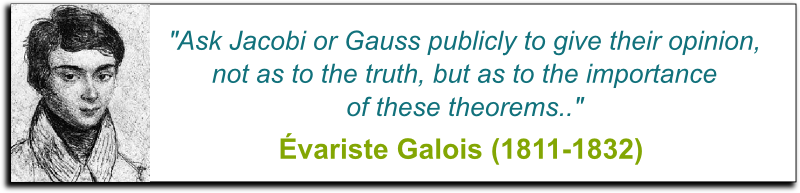Now, quite often, determining isomorphisms is beyond our reach. In particular, some objects are so complicated (like the absolute Galois group) that there may not be any well-known structure that they are isomorphic too. In such cases, it may be interesting enough to only compare them with simple structures, which can be done by morphisms. Roughly, a morphism is a way to plug into a structure into another one, while preserving the essence of the structure. In some sense, a morphism is precisely the formal way of forgetting about most of the structure. It’s algebra at its best!

Can you give an example?

For instance, we can plug the group of symmetries of an equilateral triangle into the group of numbers modulo 2, by only asking whether a symmetry involves a flip of the triangle or not.

For those familiar with group theory, what I’m hinting at here is actually the sign morphism $S_3 \rightarrow \{ \pm 1 \}$.

Nowadays, Galois’ groups are ubiquitous in modern mathematics and fundamental physics. But they’re not the most useful piece of modern algebra…

## Linear Algebra

Given their ubiquity in today’s applied mathematics, it sounds unbelievable that linear algebra was only developed in the late 19th century, with works by Cayley, Hamilton, Jordan and Peano. Before that, it was foolish to discuss spaces of dimensions 4, 5 or more…

Is it no longer the case?

While string theorists, who believe that our spacetime really has 11 spatial dimensions, struggle to have their ideas accepted, the existence (or, at least, the usefulness) of high dimension spaces becomes an obvious fact once we forget that they need to have anything to do with our space.

So how should I think about high dimension space?

Through the enlightening lenses of algebra! Descartes already paved the way for us to follow. He showed that the 1-dimension line could be described by a single variable, the 2-dimension plane by 2, and the 3-dimension space by 3.

Let me guess… Spaces of dimension $n$ can be described by $n$ variables, can’t they?

Exactly! Now, crucially, Descartes’ algebraic geometry dictionary works both ways. This means that systems of $n$ variables can be naturally embedded within $n$-dimension spaces. And this is precisely what Big Data is all about!

Can you give an example?

A major example is that of linear programming, developed by John von Neumann, Georg Dantzig and Leonid Kantorovich.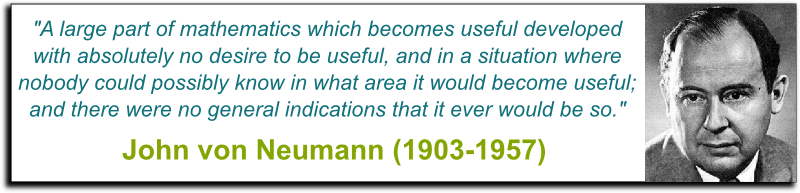It consists of optimization methods developed for such very high dimension spaces. Linear programming require a linear structure on these spaces, and it turns out that many problems already have a natural linear structure they are built in, while others can be correctly approximated by linear models. As a result, linear programming has since become a bedrock of optimization problems, and the most useful of all mathematical tools to address industrial problems.

Waw! I didn’t know that such advanced algebra could be so useful!

It is! Now, that’s pretty cool. But it’s actually no where near the actual most breathtaking application of high dimension spaces (and probably of algebra as well).

What’s this most breathtaking application?

I’m talking about quantum mechanics. Weirdly enough, as we got to study closer and closer the most elementary pieces that make up our universe, we didn’t find something simple. In fact, at its smallest scales, the world is not made of the individual elementary balls, as opposed to every textbook’s representation of atoms. This image of atoms and electrons is deeply flawed. I’d even say that any complete understanding of particle physics absolutely requires to forget about this huge misconception.

So how should we think about particles?

Instead, particles have some inner structure, which is rather complicated. Indeed, a particle cannot be described with a few numbers, as we would describe the coordinates and velocity of point-like objects. But rather, a particles must be described by vectors, whose coordinates are complex numbers, and that live in very high dimension spaces (in fact, spaces of infinite dimensions!).

But why on earth would the fundamental laws of Nature and of particles be written in the language of such an advanced form of algebra?

It’s probably one of those biggest mysteries of physics. Or maybe, at least that’s what I think as an applied mathematician, it’s due to the extreme simplicity and completeness of the beautiful structures of complex numbers and vector spaces. If you want to have a concise, appealing and powerful description of some complicated phenomenon, it seems that the best thing you can do is to model it with complete, simple and elegant structures. I’d even go as far as saying that I’m surprised not to have encountered complex numbers in fields like biology, economics and sociology (although they do make wide use of linear algebra and calculus). But I bet it’s only because mathematicians have not pondered these topics long enough yet, to apply the full power of algebra. Maybe, the next breakthroughs in these fields will require tapping further in the immense power of forgetting…

## Let’s Conclude

People often regard mathematics as too abstract to have anything to do with reality. Granted, especially when it comes to modern mathematics, that is often the case. But, amazingly, while the hugely abstract ideas that mathematicians have been developing throughout the ages usually only had aesthetic motivations, many of them ended up having deep and fundamental applications precisely to our understanding of reality. It sounds counter-intuitive to think that Al-Khwarizmi’s art of forgetting turned out to be the key to breakthroughs in our worldview. Yet, the History of algebra is precisely a cumulation of evidence for this. From Descartes’ geometry to Fermat’s arithmetics, from Gauss’ theorem to Galois’ theory, from linear programming to quantum mechanics, the realm of phenomena explained by algebra has undergone an overwhelming growth. Who knows what its limits are?

Now, unfortunately, this article is too short to mention more modern advancements in algebra. Among others, there are its application to topological shape classification, called algebraic topology, its use in quantum field theories through gauge theory and the massive 10,000-page-long classification of finite simple groups. Also, we’ve quickly mentioned Andrew Wiles’ use of elliptic curves and various number fields (and so-called modular forms) to solve Fermat’s last theorem. This result uses some basic building block of the larger Langlands program that conjectures deep connections between various fundamental algebraic structures, including the mystic Galois absolute group, under the light of so-called L-functions. This program is today’s finest piece of mathematics, and solving it (or part of it) is the challenge left to today’s greatest mathematicians.